function 订阅
Function，表示子例程的一般性名词。在某些编程语言中，它指带返回值的子例程或语句。在一些编程语言中起着关键字的作用。在Python中，function是非常重要而且常见的，一般获取类或函数的参数信息。 展开全文Function，表示子例程的一般性名词。在某些编程语言中，它指带返回值的子例程或语句。在一些编程语言中起着关键字的作用。在Python中，function是非常重要而且常见的，一般获取类或函数的参数信息。

Function

Function分类

• ## Matlab中function的用法！

万次阅读 多人点赞 2014-07-24 15:22:12
function用来定义函数，一般一个函数放在一个.m文件里。举个简单的例子建立一个myfunction.m，然后在文件中写。 function y=myfunction(a,b) 其中a,b是输入函数的参数，y是函数返回的值。当需要返回多个值时，可以...
function用来定义函数，一般一个函数放在一个.m文件里。举个简单的例子建立一个myfunction.m，然后在文件中写。
function y=myfunction(a,b)
其中a,b是输入函数的参数，y是函数返回的值。当需要返回多个值时，可以将y看作一个数组，或者直接将函数的开头写成如function [x,y]=myfunction(x,y)的形式。
然后就是定义函数的内容，通俗的说就是怎样由输入参数a,b得到返回值y。比如最简单的y=a+b。
总结一下，将下面的代码
function y=myfunction(a,b)
y=a+b;
保存为一个m文件myfunction.m，调用方式如下。
num=myfunction(3,4);
这样就可以由函数中的y=a+b得到num的值是3+4=7。

如果不明白随意去matlab下打开一个*.m的文件看一下就OK了！

展开全文• ## Matlab S-function 使用总结

万次阅读 多人点赞 2019-05-24 09:45:03
        近期在学习电机的智能控制，里面用到了Matlab中的S函数，在此先对S-function做一个小结。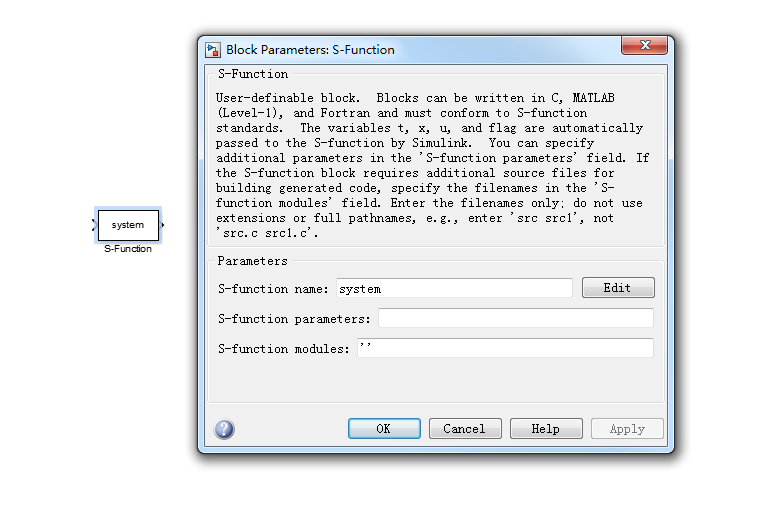S-function name:填入S-function的函数名称，这样就建立了S-function模块与M文件形式的S-function之间的对应关系，单机后面的Edit可以打开S-function的M文件的编辑窗口。

S-function parameters：填入S-function需要输入的外部参数的名称，如果有多个变量的话，中间用逗号隔开，如a,b,c.

S-function modules:只有S-function是用C语言编写并用MEX工具编译的C MEX文件时，才需要填写该参数。

设置完这些参数后，S-function模块就成为了一个具有指定功能的模块，它的功能取决于S-function的内容，也可对内容修改进而改变模块功能。

编写M文件S-function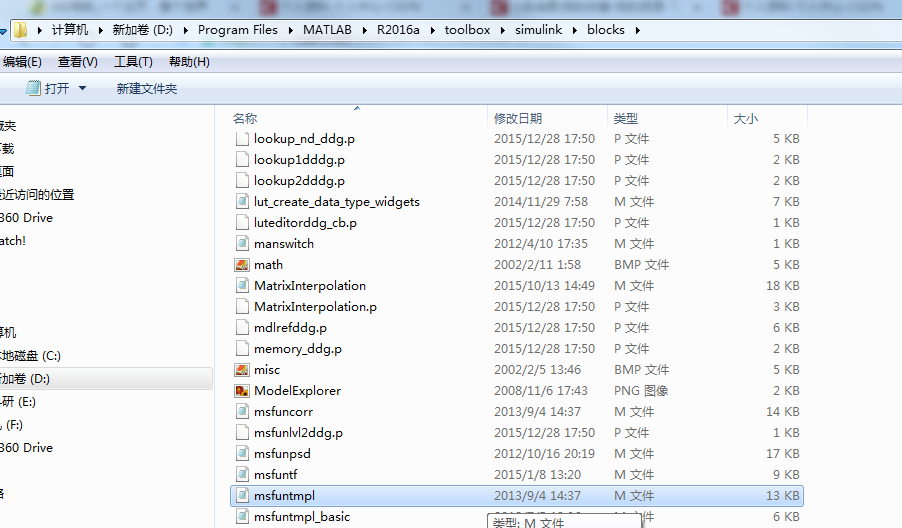该模板由一个主函数和六个子函数组成，每个子函数对应一个特定的flag值。主函数通过flag的值分别调用不同的子函数。在仿真期间，这些子函数被S-function以回调程序的方式调用，执行S-function所需的任务。

学习S-function最直接有效的方法就是学习S-function范例，下面是我详细分析的sfuntmpl.m模板代码内容：

function [sys,x0,str,ts,simStateCompliance] = sfuntmpl(t,x,u,flag)
%主函数
%主函数包含四个输出：
%                 sys数组包含某个子函数返回的值
%                 x0为所有状态的初始化向量
%                 str是保留参数，总是一个空矩阵
%                 Ts返回系统采样时间
%函数的四个输入分别为采样时间t、状态x、输入u和仿真流程控制标志变量flag
%输入参数后面还可以接续一系列的附带参数simStateCompliance
switch flag,
case 0,
[sys,x0,str,ts,simStateCompliance]=mdlInitializeSizes;
case 1,
sys=mdlDerivatives(t,x,u);
case 2,
sys=mdlUpdate(t,x,u);
case 3,
sys=mdlOutputs(t,x,u);
case 4,
sys=mdlGetTimeOfNextVarHit(t,x,u);
case 9,
sys=mdlTerminate(t,x,u);
otherwise

end
%主函数结束
%下面是各个子函数，即各个回调过程
function [sys,x0,str,ts,simStateCompliance]=mdlInitializeSizes
%初始化回调子函数
%提供状态、输入、输出、采样时间数目和初始状态的值
%初始化阶段，标志变量flag首先被置为0，S-function首次被调用时
%该子函数首先被调用，且为S-function模块提供下面信息
%该子函数必须存在
sizes = simsizes;
%生成sizes数据结构，信息被包含在其中
sizes.NumContStates  = 0;
%连续状态数，缺省为0
sizes.NumDiscStates  = 0;
%离散状态数，缺省为0
sizes.NumOutputs     = 0;
%输出个数，缺省为0
sizes.NumInputs      = 0;
%输入个数，缺省为0
sizes.DirFeedthrough = 1;
%是否存在直馈通道，1存在，0不存在
sizes.NumSampleTimes = 1;
%采样时间个数，至少是一个
sys = simsizes(sizes);
%返回size数据结构所包含的信息
x0  = [];
%设置初始状态
str = [];
%保留变量置空
ts  = [0 0];
%设置采样时间
simStateCompliance = 'UnknownSimState';
function sys=mdlDerivatives(t,x,u)
%计算导数回调子函数
%给定t,x,u计算连续状态的导数，可以在此给出系统的连续状态方程
%该子函数可以不存在
sys = [];
%sys表示状态导数，即dx
function sys=mdlUpdate(t,x,u)
%状态更新回调子函数
%给定t、x、u计算离散状态的更新
%每个仿真步内必然调用该子函数，不论是否有意义
%除了在此描述系统的离散状态方程外，还可以在此添加其他每个仿真步内都必须执行的代码
sys = [];
%sys表示下一个离散状态，即x(k+1)
function sys=mdlOutputs(t,x,u)
%计算输出回调函数
%给定t,x,u计算输出，可以在此描述系统的输出方程
%该子函数必须存在
sys = [];
%sys表示输出，即y
function sys=mdlGetTimeOfNextVarHit(t,x,u)
%计算下一个采样时间
%仅在系统是变采样时间系统时调用
sampleTime = 1;
%设置下一次采样时间是在1s以后
sys = t + sampleTime;
%sys表示下一个采样时间点
function sys=mdlTerminate(t,x,u)
%仿真结束时要调用的回调函数
%在仿真结束时，可以在此完成仿真结束所需的必要工作
sys = [];

S-function应用实例

用S-function实现Gain增益模块，即对输入信号进行放大。

(1)首先将模板文件sfuntmpl.m另存为Gain.m,修改主函数，增加新的参数gain,修改函数名为Gain: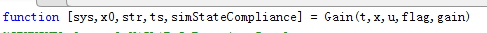(2)增益参数是用来计算输出值的，所以需要对mdlOutput的调用进行修改：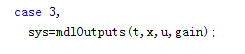(3)修改mdlInitializeSizes初始化回调子函数：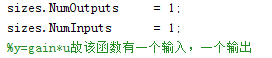(4)修改mdlOutput输出子函数：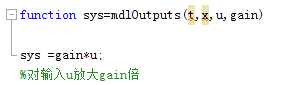(5)保存Gain.m，并建立如下图所示的系统模型，对余弦信号的幅值放大gain倍。在S-function模块的参数对话框中设置S-function parameter 为3（该设置即为外部输入gain的大小）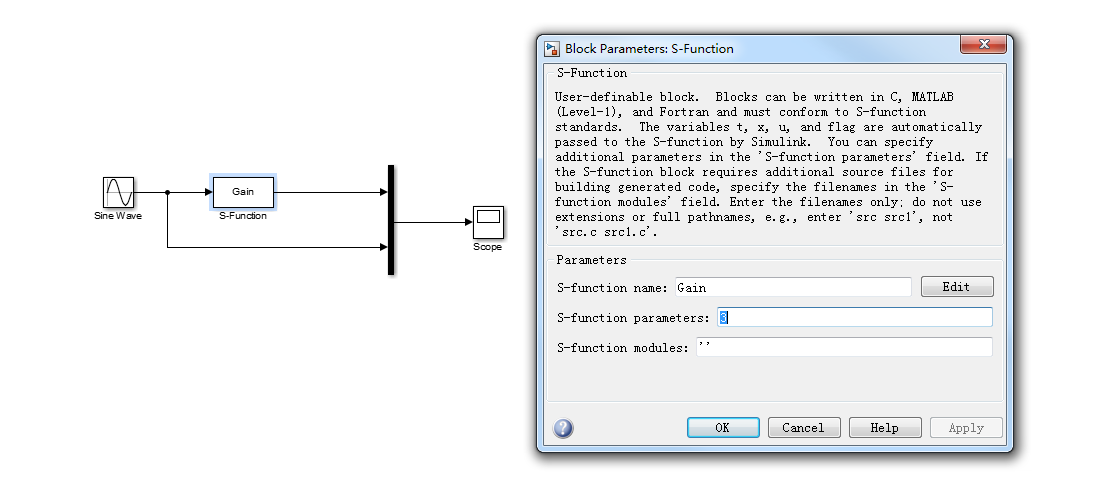完整代码如下所示：

function [sys,x0,str,ts,simStateCompliance] = Gain(t,x,u,flag,gain)
switch flag,
case 0,
[sys,x0,str,ts,simStateCompliance]=mdlInitializeSizes;
case 1,
sys=mdlDerivatives(t,x,u);
case 2,
sys=mdlUpdate(t,x,u);
case 3,
sys=mdlOutputs(t,x,u,gain);
case 4,
sys=mdlGetTimeOfNextVarHit(t,x,u);
case 9,
sys=mdlTerminate(t,x,u);
otherwise
end
function [sys,x0,str,ts,simStateCompliance]=mdlInitializeSizes
sizes = simsizes;
sizes.NumContStates  = 0;
sizes.NumDiscStates  = 0;
sizes.NumOutputs     = 1;
sizes.NumInputs      = 1;
sizes.DirFeedthrough = 1;
sizes.NumSampleTimes = 1;
sys = simsizes(sizes);
x0  = [];
str = [];
ts  = [0 0];
simStateCompliance = 'UnknownSimState';
function sys=mdlDerivatives(t,x,u)
sys = [];
function sys=mdlUpdate(t,x,u)
sys = [];
function sys=mdlOutputs(t,x,u,gain)
sys =gain*u;
function sys=mdlGetTimeOfNextVarHit(t,x,u)
sampleTime = 1;
sys = t + sampleTime;
function sys=mdlTerminate(t,x,u)
sys = [];

(6)运行仿真，仿真结果如下图所示蓝线为输入，黄线为输出，输出放大了3（gain）倍。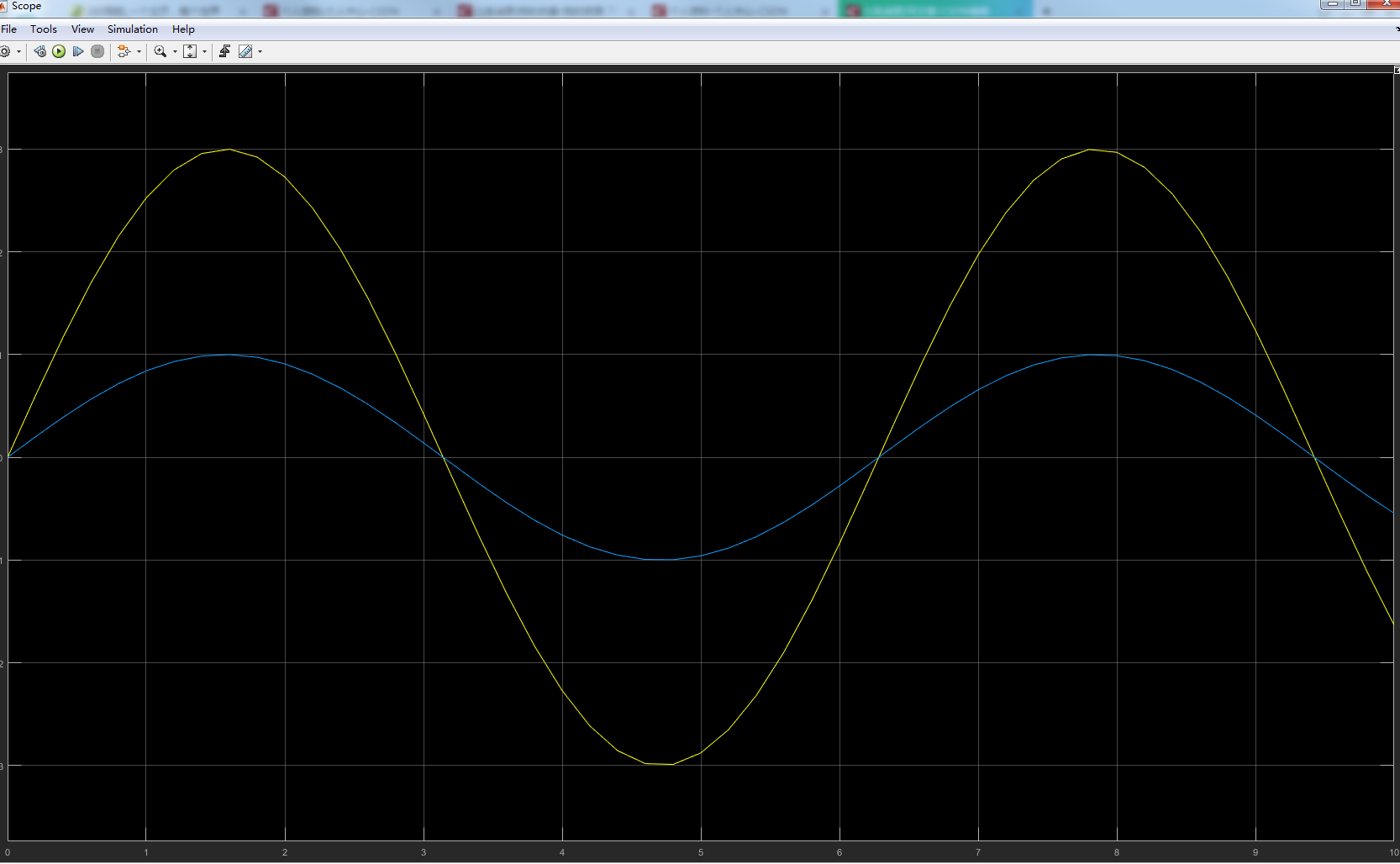注：在参数gain的设置时，为了更直观的表达可以对S-function模块进行封装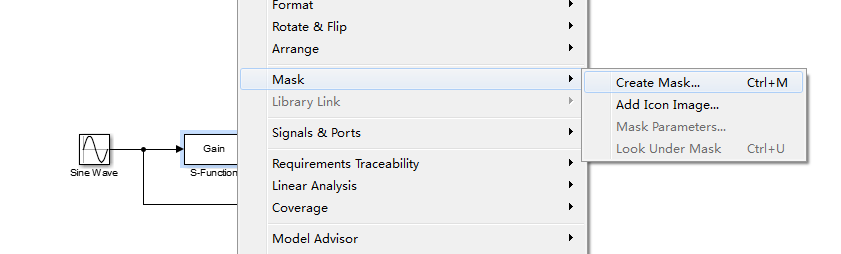进行参数设置，如下图所示：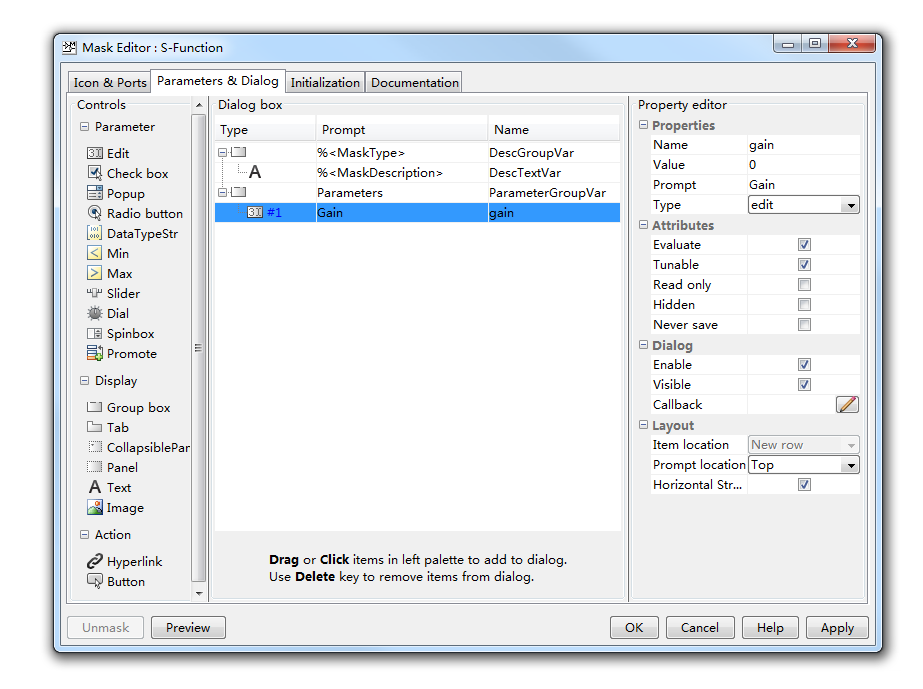对S-function的S-function parameter 选项重新设置，如下图所示：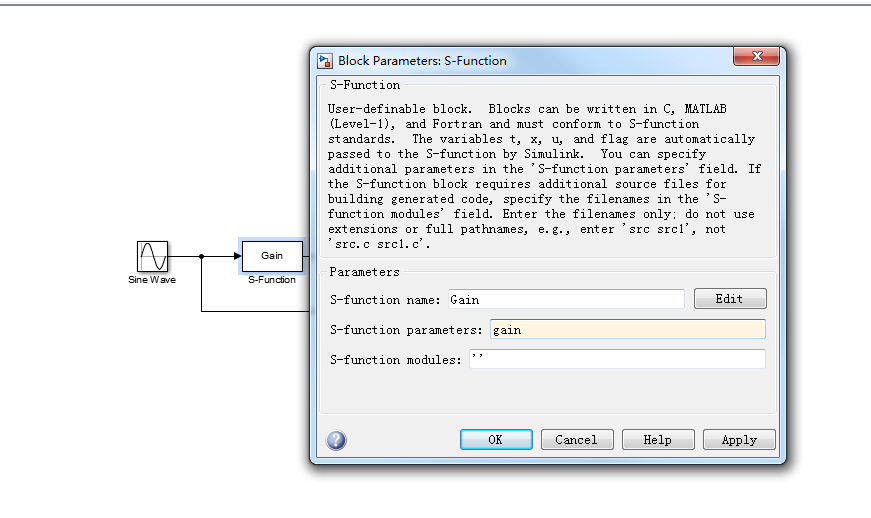S-function parameter 的名称要和封装模块中的参数名称一样，此例中都为gain。都设置完成后再次点击S-function函数Gain会弹出如下参数设置框：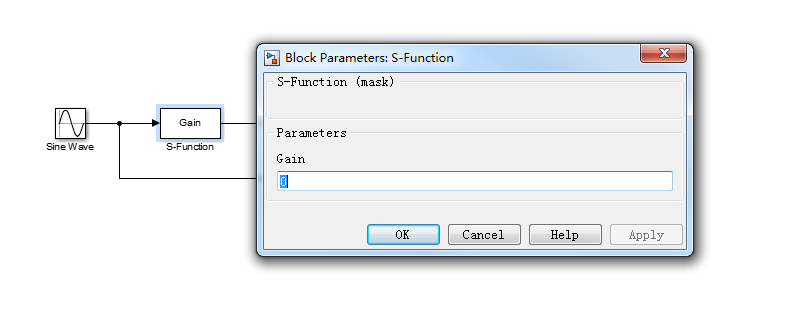把增益gain设置为5，仿真结果如下：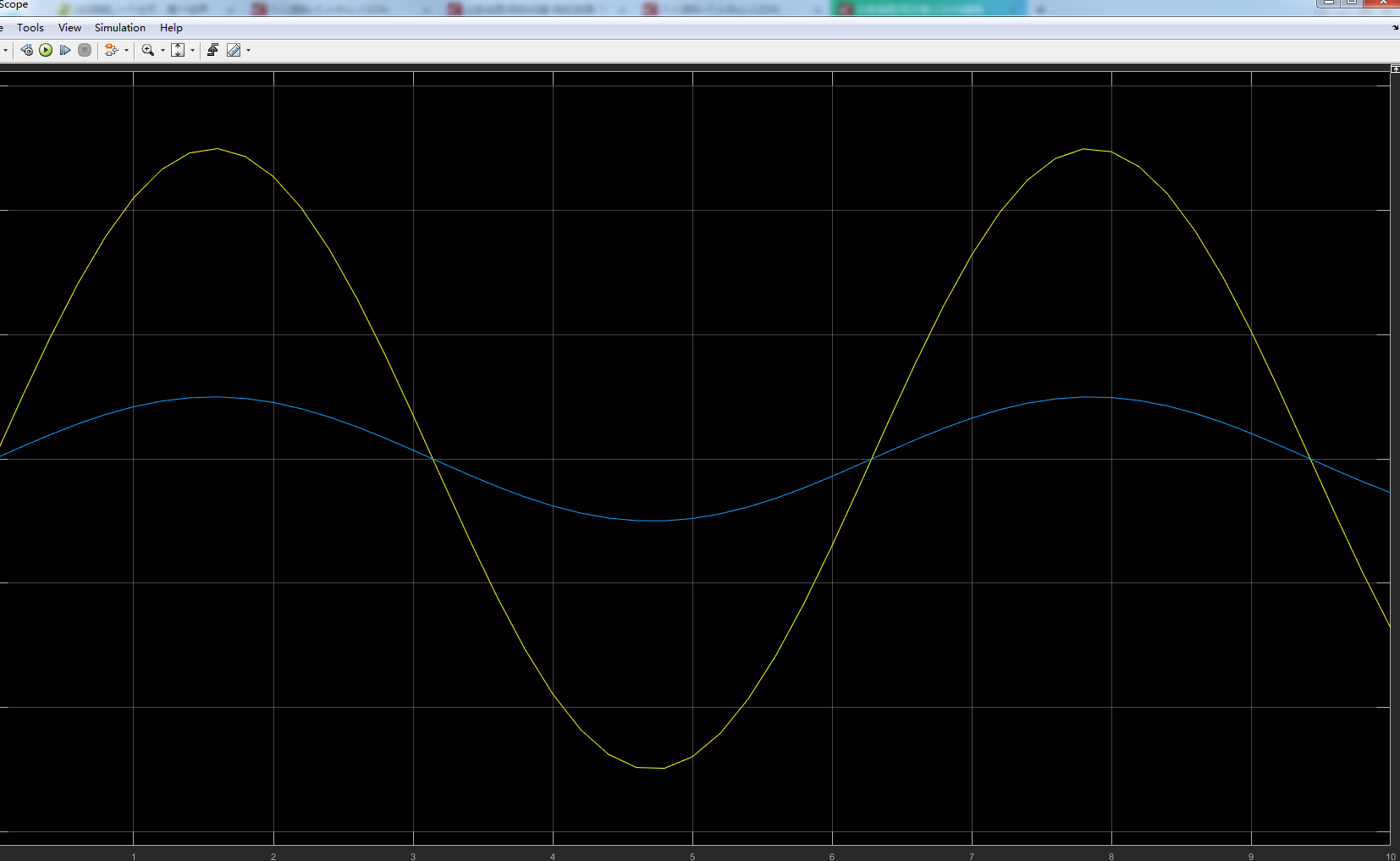这样可以更方便的对增益参数gain进行设置。


展开全文S函数编程
• 图左模块以前叫 Embedded MATLAB Function，从2011a改名为 MATLAB Function。 两种模块均只支持单输入单输出的函数构型， 且 Embedded MATLAB Function 模块，比起普通的MATLAB语法要严格，导致部分MATLAB函数无法...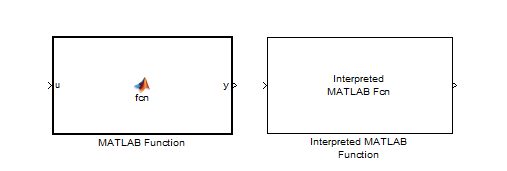图左模块以前叫 Embedded MATLAB Function，从2011a改名为 MATLAB Function。
两种模块均只支持单输入单输出的函数构型，
且 Embedded MATLAB Function 模块，比起普通的MATLAB语法要严格，导致部分MATLAB函数无法使用，例如无法使用syms定义符号变量，但好处是不需要m文件配合，双击即可看到代码。而Interpreted MATLAB Function 与常规m函数一致，但也须做到但输入单输出。
Interpreted MATLAB Function 输出为按列排布的向量，无法输出矩阵！！


展开全文函数
• Error: File: D:\matlab\work\huitu.m Line: 6 Column: 7 Missing variable or function. 这个错误如果没有找对解决办法还是会很浪费时间的，这个错误其实就是检查一下代码中的所有的标点符号，尤其是引号...
??? Error: File: D:\matlab\work\huitu.m Line: 6 Column: 7 Missing variable or function.

这个错误如果没有找对解决办法还是会很浪费时间的，这个错误其实就是检查一下代码中的所有的标点符号，尤其是引号是不是英文格式的，可以把输入法切换成英文的
然后再把所有的引号重新输入一遍就会解决。

展开全文输入法
• ## Matlab自定义函数

千次阅读 2015-06-30 11:03:57
Matlab提供了强大的函数库供用户调用，但也支持用户自己定义函数。本文使用了范德堡大学教授Akos Ledeczi授课中的例子来一步步说明如何在Matlab中自定义函数。...function myRand a = rand(3,4) end
• ## Matlab 基于svm的图像物体分类

万次阅读 热门讨论 2018-05-16 20:27:10
本周工作日志，老师布置了一个小作业，让我们使用matlab实现图像物体分类 matlab 图像分类 1分类原理 基于一个很朴素的观点，不同物体在色素三维空间里的分布不同，使用svm可以构造一个...function showline(i...图像分类 svm
• ## BP神经网络的MATLAB实现

万次阅读 多人点赞 2017-05-18 15:56:04
算法流程关于BP神经网络的公式推导，上一篇博文《BP神经网络原理简单介绍以及公式推导（矩阵形式和分量形式） 》已经做了详细的说明。接下来，我们利用MATLAB对BP...sigmoid.mfunction [ out ] = sigmoid( in ) %SIGM神经网络
• Matlab function是一支持使用M语言编写模块功能，并能够将其生成C代码，用于开发桌面应用和嵌入式应用模块。 在function 头的下一行增加%#codegen符号，其作用是为了使静态代码分析器Code Analyzer 诊断代码并提示...simulink
• ## matlabfunction的使用

万次阅读 多人点赞 2017-11-12 10:19:29
Matlab函数定义格式，用关键字function定义函数   function [输出变量] = 函数名称(输入变量） % 注释 % 函数体   简单的函数如下：   function [a,b,c]=test(d,e,f) a=d+e; b=e+f; c=f+d; end   ...
• BPSK调制  ...function [y]=modbpsk(x) mapping=[1 -1]; y=mapping(x(:,:)+1); QPSK调制  %此段代码实现信号的QPSK调制 %输入x为0 1 2 3 输出y为1-1i -1+1i 1+1i -1-1i function [y]=modqpsk(x) ...
• ## Matlabfunction函数如何使用

万次阅读 多人点赞 2019-06-28 14:16:41
1、创建.m文件，.m文件中用关键字function定义函数，定义函数的格式如下： function [输出变量] = 函数名称(输入变量） 输入变量和输出变量可以是一个，也可以是多个。 function [输出变量] = 函数名称(输入变量） %...
• ## Embedded MATLABFunction

千次阅读 2011-01-07 11:45:00
function y = fcn(u) y=0;% 因为是函数，所以对输出初始化 if u==1  y=0; elseif u==0  y=1; end   如果不对输出初始化，即函数值无法计算，会产生如下错误：y is...
• ## matlab callback function

千次阅读 2016-11-08 14:08:33
'Callback', {@setPointNum,TMsubsub} 'Callback', @setPointNum
• function用来定义函数，一般一个函数放在一个.m文件里。举个简单的例子建立一个myfunction.m，然后在文件中写。 function y=myfunction(a,b) 其中a,b是输入函数的参数，y是函数返回的值。当需要返回多个值时，可以...
• 1、BPSK 目录 1、BPSK 2、QPSK 3、MPSK 4、QAM 相位状态表 表 1.1 BPSK相位状态表 ... BPSK在AWGN信道中能够获得最好的误码率性能，频谱效率：1bps/Hz. ...function [mod_symbol] = bpskmod(bit_in)...
• 这个程序直接运行为什么在命令窗口直接输出的是 MyAdd ans = 3 而不是c=3,d=4呢？怎样才能直接运行出现我希望的结果呢？谢谢各位 function [c d] = MyAdd(a, b); a=1; b=2; c = a + b; d=a+c; end
• As previous metioned that I install the matlab on our linux workstation and last night & this morning just write matlab code to give an edge of an imagefrist learn the help file in the matlabIts easycommand linux file
• 这时，可以先用符号函数 int 表示积分，然后用matlabFunction 将符号函数转化为匿名函数，从而达到化简目的。 例如，有如下函数： 其中，f(z) 为标准正态分布的密度函数，这个函数是一个关于 x 的函数，但...matlab 正态分布积分
• ## Matlab S-Function详解

万次阅读 多人点赞 2017-10-24 22:00:51
Part I: ... s函数可以用matlab、C、C++、Fortran、Ada等语言来写， 这儿我只介绍怎样用matlab语言来写吧（主要是它比较简单） < xmlnamespace prefix ="o" ns ="urn:schemas-microsoft-com:offic
• 标题中的例子只是一个参考，这里主要是运用ezplot函数画图 例如：分别画函数： (x-2.7)*(0.9*(x-2.7)-0.2*(y-1.6))+(y-1.6)*(-0.2*(x-2.7...function [z1,z2]=huatu() z1=ezplot('(x-2.7)*(0.9*(x-2.7)-0.2*(y-1.6
• ## MATLAB中调用eemd函数

千次阅读 多人点赞 2019-07-16 15:18:06
#MATLAB 中调用EEMD 函数 一般情况添加eemd.m和extrema.m到主函数的同一个文件夹就可直接调用了。 eemd.m中是英文注释，解释该函数各个参量的意义以及如何取值。 function allmode=eemd(Y,Nstd,NE) Y为输入，待分解...
• Matlab：成功解决Undefined function or variable "B" 目录 解决问题 解决方法 解决问题 Undefined function or variable "B" 解决方法 变量B没有被定义或者该函数没有定义，先去工作空间查看有...函数句柄plot vector